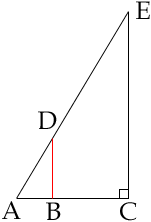Hello!
My name is Laura. I am currently a sophomore in high school. (student). I have two math problems that are difficult for me. I was wondering if you help me solve these problems for me. Also, can you please show me all work!

Thanks so much for all your time and effort!

A lamppost line EC casts a shadow line AC. A 30 cm ruler line DB has been moved from A so that it's shadow falls just within the shadow of the lamppost.

1. Suppose the length of the ruler's shadow is 42 cm. What is the slope of the imaginary line AE?

2. Suppose the lamppost's shadow is 15 m. long. How tall is the lamppost?
Hi Laura,
 For the first problem the slope of a line is "the rise over the run". Here you know that |DB| = 30 cm (this is the rise) and |AB| = 42 cm (this is the run) and hence the slope is 30/42 = 5/7. For the second problem you can use the fact that the triangles ACE and ABD are similar, and hence the ratios of the length of the corresponding sides are equal. That is |AC|/|AB| = |CE|/|BD|. Before you do any calculations make sure that you have all the measurements in the same units.Cheers,
Penny
Go to Math Central